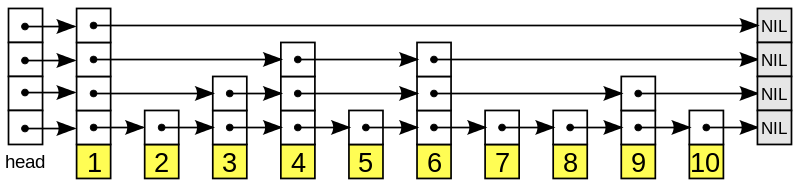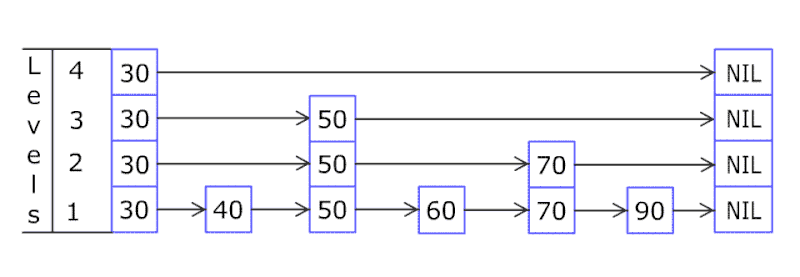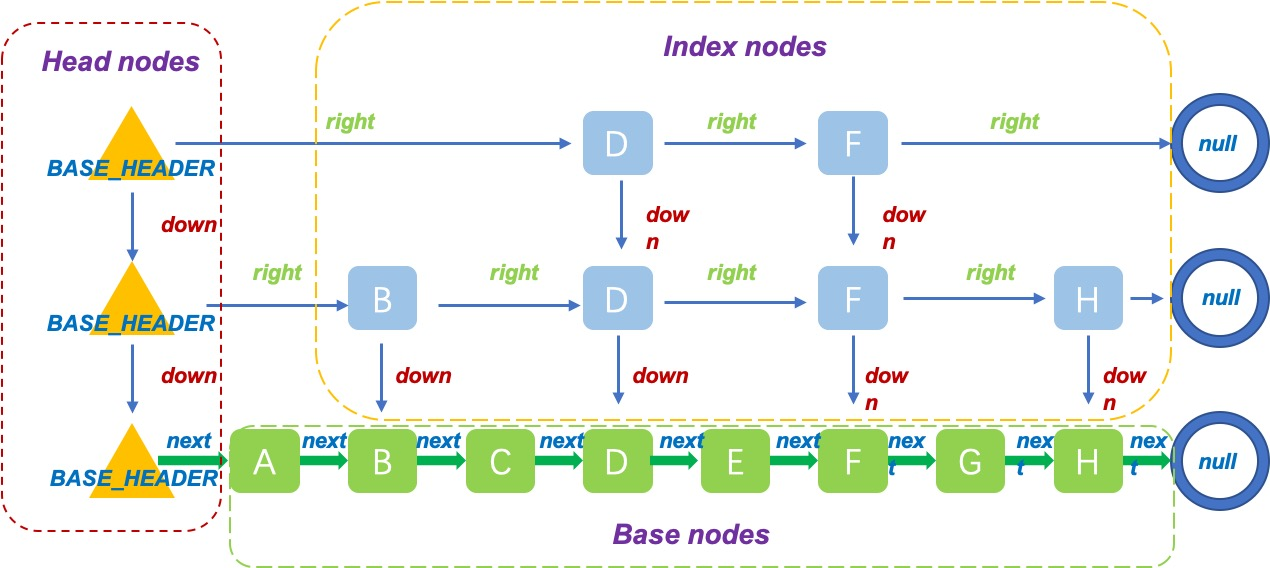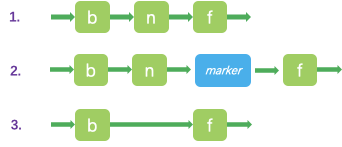# SkipList和java中ConcurrentSkipListMap的实现

SkipList和java中ConcurrentSkipListMap的实现

# SkipList# ConcurrentSkipListMap

ConcurrentSkipListMap是一个并发的SkipList，那么它具有两个特点，SkipList和concurrent。我们分别来讲解。

## SkipList的实现base nodes组成了有序的链表结构，是ConcurrentSkipListMap的最底层实现。

``````    static final class Node<K,V> {
final K key;
volatile Object value;
volatile Node<K,V> next;

/**
* Creates a new regular node.
*/
Node(K key, Object value, Node<K,V> next) {
this.key = key;
this.value = value;
this.next = next;
}
}
``````

index nodes是构建SkipList上层结构的基本节点：

``````    static class Index<K,V> {
final Node<K,V> node;
final Index<K,V> down;
volatile Index<K,V> right;

/**
* Creates index node with given values.
*/
Index(Node<K,V> node, Index<K,V> down, Index<K,V> right) {
this.node = node;
this.down = down;
this.right = right;
}
}
``````

``````    static final class HeadIndex<K,V> extends Index<K,V> {
final int level;
HeadIndex(Node<K,V> node, Index<K,V> down, Index<K,V> right, int level) {
super(node, down, right);
this.level = level;
}
}
``````

``````head = new HeadIndex<K,V>(new Node<K,V>(null, BASE_HEADER, null),null, null, 1);
``````

## concurrent的实现``````        Node(Node<K,V> next) {
this.key = null;
this.value = this;
this.next = next;
}
``````